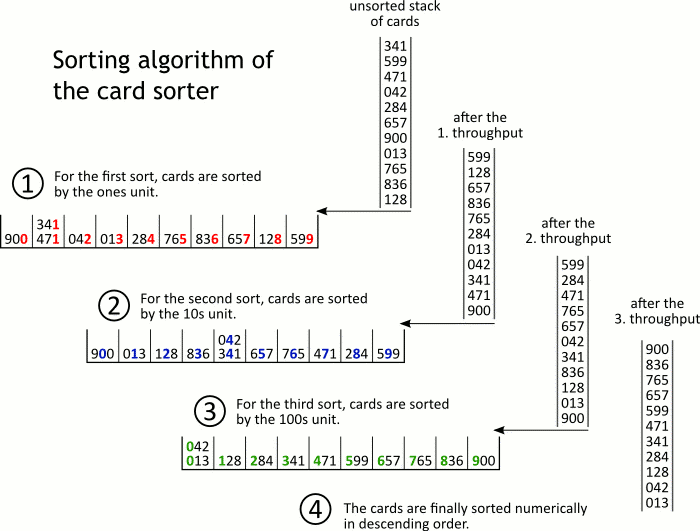## The function of the punch card sorter

Only people who are born prior to 1960 may have knowledge of the punch card machines. We would like to give you an example of these historic machines' functionality.

If we had a list of customer names to be sorted, we would first give each customer a three digit customer number. We can then sort this list in numerical (ascending) order or sort by their names.

If we would sort the customer names manually, the cards would initially be sorted by the 100's decimal place into 10 piles. Subsequently each of the 10 piles would be sorted by the 10's decimal place into 10 piles. Finally each of the piles would be sorted by the 1's decimal place.Machine sorting can not use this procedure since we would need an unlimited number of sorting compartments (pockets). Thus it sorts the other way around, from the 1's decimal place to the 100's decimal place. In summary, in each step (2-4, according to the diagram), there would be a maximum of only 10 card decks per sort.

Summing up, this yields the basic rules of mechanical sorting, as the punch card collator uses them:

• The elements which have to be sorted are treated as decimal numbers, each number is broken down in its decimal places
• The sorting algorithm starts with the least significant digit and ends with the most significant one
• The sorting algorithm needs as many loops as the number of digits in each number (three loops in this case)